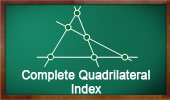# Online Geometry: Complete Quadrilateral - Table of ContentGeometry Problem 1462.
Newton-Line, Newton-Gauss Line, Complete Quadrilateral, Midpoints of Sides and Diagonals, Collinear Points.

Dynamic Geometry 1460.
Newton-Gauss Line, Complete Quadrilateral, Midpoints of Diagonals, Collinear Points, Step-by-step Illustration using GeoGebra.

Dynamic Geometry 1450.
Ortholine, Steiner Line, Complete Quadrilateral, Collineal Orthocenters. Step-by-step illustration using GeoGebra.

Step-by-Step construction, Manipulation, and animation
TracenPoche Interactive Geometry Software.

Newton's Theorem, Newton-Gauss Line
Complete quadrilateral theorem. Using TracenPoche Tracenpoche Interactive Geometry Software
, Online Step-by-Step construction, manipulation, and animation.

Geometry Problem 1169
Complete Quadrilateral, Orthocenters of the Component Triangles, Collinear Points, Ortholine, Steiner-line, Orthocentric line.

Geometry Problem 750.
Complete Quadrilateral, Triangle, Diagonal, Parallel, Similarity, Metric Relations.

Geometry Problem 748.

Problem 564.
Complete Quadrilateral, Incenter, Excenter, Concyclic Points, Incircle, Excircle.

Problem 548.
Triangle, Transversal, Four Circumcircles, Circumcenters, Concyclic Points, Similarity, Complete Quadrilateral.

Problem 547.
Triangle, Transversal, Four Circumcircles, Concurrency, Complete Quadrilateral.

Proposed Problem 447.